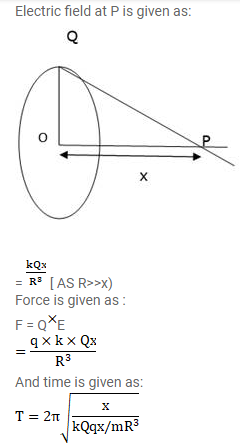# Positive charge Q is distributed uniformly over a circulating of`
Question:

Positive charge $Q$ is distributed uniformly over a circulating of radius $\mathrm{R}$. A particle having a mass $\mathrm{m}$ and a negative charge $q$, is placed on its axis at a distance $x$ from the centre. Find the force on the particle. Assuming $x Solution:$\mathrm{T}=\sqrt{\frac{16 \pi^{3} e_{0} \mathrm{mR}}{\mathrm{qQ}}}\$